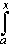#Interactive Real Analysis

Next | Previous | Glossary | Map

## 7.1. Riemann Integral

### Corollary 7.1.20: Integral Evaluation Shortcut

Suppose f is an continuous function defined on the closed, bounded interval [a, b], and G is a function on [a, b] such that G'(x) = f(x) for all x in (a, b). Thenf(x) dx = G(b) - G(a)
Note: The function G is often called Antiderivative of f, and this corollary is called First Fundamental Theorem of Calculus in many calculus text books. Those books also define a Second Fundamental Theorem of Calculus, which we called the Fundamental Theorem of Calculus.

### Proof:

This being a corollary means that it must be easy to prove. We already know from the previous theorem that if we define the function
F(x) =f(t) dt
then F(b) - F(a) =f(x) dx and F' = f. What we need to prove is that if we take any function G such that G'(x) = f(x) then G(b) - G(a) =f(x) dx also. So, define
H(x) = F(x) - G(x)
where F and G are as defined above. Then
H'(x) = F'(x) - G'(x) = f(x) - f(x) = 0
so that H(x) = c for some constant c. But then F(x) = G(x) + c so that
F(b) - F(a) = (G(a) + c) - (G(b) + c) = G(b) - G(a)
Next | Previous | Glossary | Map